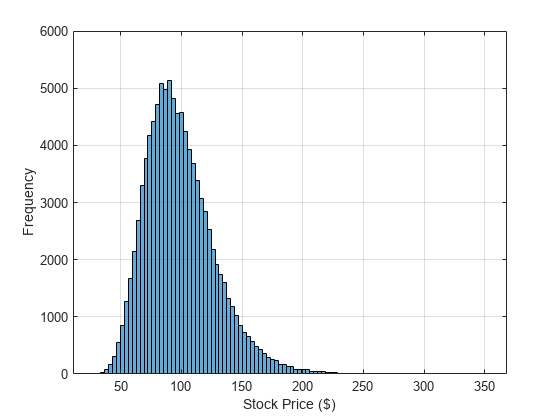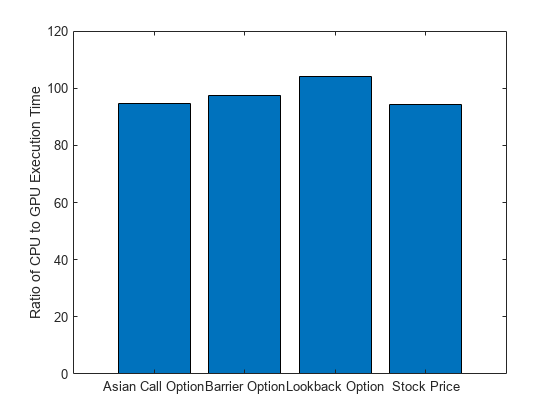# Using GPU `arrayfun` for Monte-Carlo Simulations

This example shows how to calculate prices for financial options on a GPU using Monte-Carlo methods.

The example uses three simple types of exotic option, but you can price more complex options in a similar way. In this example, you compare the time taken to run Monte-Carlo Simulations on the CPU and using `arrayfun` on the GPU.

### Stock Price Evolution

Assume that prices evolve according to a log-normal distribution related to the risk-free interest rate, the dividend yield (if any), and the volatility in the market. Further, assume that all these quantities remain fixed over the lifetime of the option. These assumptions lead to this stochastic differential equation for the price.

$dS=S×\left[\left(r-d\right)dt+\sigma ϵ\sqrt{dt}\right]$,

where $S$ is the stock price, $r$ is the risk-free interest rate, $d$ is the annual dividend yield of the stocks, $\sigma$ is the volatility of the price, and $ϵ$ represents a Gaussian white-noise process. Assuming that $\left(S+\Delta S\right)/S$ is log-normally distributed, this differential equation can be discretized to obtain this equation.

${S}_{t+1}={S}_{t}×\mathrm{exp}\left[\left(r-d-\frac{1}{2}{\sigma }^{2}\right)\Delta t+\sigma ϵ\sqrt{\Delta t}\right]$.

Examine a two-year time window using \$100 of stock with these assumptions:

• The stocks yield a 1% dividend each year.

• The risk-free government interest rate is 0.5%.

• The price is sampled daily, with 250 working days per year.

• The market volatility is 20% per annum.

```stockPrice = 100; timeToExpiry = 2; dividend = 0.01; riskFreeRate = 0.005; sampleRate = 1/250; volatility = 0.20;```

To ensure predictable results, set the seed for the CPU and GPU random number generators.

```seed = 1234; rng(seed); gpurng(seed);```

Simulate the path of the stock price over time and plot the results.

```price = stockPrice; time = 0; h = animatedline(Marker="."); while time < timeToExpiry time = time + sampleRate; drift = (riskFreeRate - dividend - volatility*volatility/2)*sampleRate; perturbation = volatility*sqrt(sampleRate)*randn; price = price*exp(drift + perturbation); addpoints(h,time,price); end grid on axis tight xlabel("Time (years)") ylabel("Stock Price (\$)")```### Time Execution on the CPU and the GPU

The `simulateStockPrice` function, provided at the end of this example, simulates the stock price using the discretized differential equation described in the previous section.

Prepare the input data for running 100,000 Monte-Carlo simulations of the stock price.

```N = 100000; startStockPrices = stockPrice*ones(N,1);```

Time 100,000 simulations on the CPU.

```tic finalStockPricesCPU = zeros(N,1); for i = 1:N finalStockPricesCPU(i) = simulateStockPrice(startStockPrices(i), ... riskFreeRate,dividend,volatility, ... timeToExpiry,sampleRate); end timeCPU = toc;```

Because each simulation gives an independent estimate of the option price, take the mean as the result.

```fprintf("Calculated average price of \$%1.4f on the CPU in %1.3f secs.\n", ... mean(finalStockPricesCPU),timeCPU);```
```Calculated average price of \$99.0857 on the CPU in 2.206 secs. ```

To run the simulations on the GPU, prepare the input data on the GPU by creating a `gpuArray` object.

`gpuStartStockPrices = gpuArray(startStockPrices);`

When you call `arrayfun` with a GPU array and a function handle as inputs, `arrayfun` applies the function you specify to each element of the array. This behaviour means that looping over each starting stock price is not necessary. The `arrayfun` function on the GPU turns an element-wise MATLAB® function into a custom CUDA® kernel, which reduces the overhead of performing the operation.

Run the `simulateStockPrice` function using `arrayfun` and time 100,000 simulations on the GPU using `gputimeit`.

```finalStockPricesGPU = arrayfun(@simulateStockPrice, ... gpuStartStockPrices,riskFreeRate,dividend,volatility, ... timeToExpiry,sampleRate); timeGPU = gputimeit(@() arrayfun(@simulateStockPrice, ... gpuStartStockPrices,riskFreeRate,dividend,volatility, ... timeToExpiry,sampleRate)); fprintf("Calculated average price of \$%1.4f on the GPU in %1.3f secs.\n", ... mean(finalStockPricesGPU),timeGPU);```
```Calculated average price of \$99.0442 on the GPU in 0.023 secs. ```

Plot the results of the Monte-Carlo simulations on the GPU in a histogram.

```histogram(finalStockPricesGPU,100); xlabel("Stock Price (\$)") ylabel("Frequency") grid on```### Pricing an Asian Option

Use a European Asian Option based on the arithmetic mean of the stock price during the lifetime of the option. The `asianCallOption` function calculates the mean price by accumulating the price during the simulation. For a call option, the function exercises the option if the average price is above the strike price. The payout is the difference between the average price and the strike price. Use the `asianCallOption`, provided at the end of this example, to simulate an Asian call option.

Set the strike price for the option to \$95.

`strike = 95;`

Time 100,000 simulations on the CPU and on the GPU using `arrayfun` and show the results.

```tic optionPricesCPU = zeros(N,1); for i=1:N optionPricesCPU(i) = asianCallOption(startStockPrices(i), ... riskFreeRate,dividend,volatility,strike, ... timeToExpiry,sampleRate); end timeAsianOptionCPU = toc; fprintf("Calculated average price of \$%1.4f on the CPU in %1.3f secs.\n", ... mean(optionPricesCPU),timeAsianOptionCPU);```
```Calculated average price of \$8.6733 on the CPU in 2.146 secs. ```
```optionPricesGPU = arrayfun( @asianCallOption, ... gpuStartStockPrices,riskFreeRate,dividend,volatility,strike, ... timeToExpiry,sampleRate ); timeAsianOptionGPU = gputimeit(@() arrayfun( @asianCallOption, ... gpuStartStockPrices,riskFreeRate,dividend,volatility,strike, ... timeToExpiry,sampleRate)); fprintf("Calculated average price of \$%1.4f on the GPU in %1.3f secs.\n", ... mean(optionPricesGPU),timeAsianOptionGPU );```
```Calculated average price of \$8.7448 on the GPU in 0.023 secs. ```

### Pricing a Lookback Option

Use a European-style lookback option whose payout is the difference between the minimum stock price and the final stock price over the lifetime of the option. The strike price for the option is the minimum stock price. Because the final stock price is always greater than or equal to the minimum, the option is always exercised and is not really optional. Use the `lookbackCallOption`, provided at the end of this example, for simulating a European-style lookback call option.

Time 100,000 simulations on both the CPU and on the GPU using `arrayfun` and show the results.

```tic optionPricesCPU = zeros(N,1); for i=1:N optionPricesCPU(i) = lookbackCallOption(startStockPrices(i), ... riskFreeRate,dividend,volatility, ... timeToExpiry,sampleRate); end timeLookbackOptionCPU = toc; fprintf("Calculated average price of \$%1.4f on the CPU in %1.3f secs.\n", ... mean(optionPricesCPU),timeLookbackOptionCPU);```
```Calculated average price of \$19.2456 on the CPU in 2.201 secs. ```
```optionPricesGPU = arrayfun(@lookbackCallOption, ... gpuStartStockPrices,riskFreeRate,dividend,volatility, ... timeToExpiry,sampleRate); timeLookbackOptionGPU = gputimeit(@() arrayfun(@lookbackCallOption, ... gpuStartStockPrices,riskFreeRate,dividend,volatility, ... timeToExpiry,sampleRate)); fprintf("Calculated average price of \$%1.4f on the GPU in %1.3f secs.\n", ... mean(optionPricesGPU),timeLookbackOptionGPU);```
```Calculated average price of \$19.3893 on the GPU in 0.021 secs. ```

### Pricing a Barrier Option

Use an up-and-out barrier option, which becomes invalid if the stock price reaches the barrier level. If the stock price stays below the barrier level, use the final stock price in a normal European call option calculation. Use the `upAndOutCallOption` function, provided at the end of this example, to simulate an up-and-out barrier call option.

Set the strike price for the option and the barrier price at which it becomes invalid. Use a strike price of \$95 and a barrier price of \$150.

```strike = 95; barrier = 150;```

Time 100,000 simulations on the CPU and on the GPU using `arrayfun` and show the results.

```tic optionPricesCPU = zeros(N,1); for i=1:N optionPricesCPU(i) = upAndOutCallOption(startStockPrices(i), ... riskFreeRate,dividend,volatility,strike, ... barrier,timeToExpiry,sampleRate); end timeBarrierOptionCPU = toc; fprintf("Calculated average price of \$%1.4f on the CPU in %1.3f secs.\n", ... mean(optionPricesCPU),timeBarrierOptionCPU);```
```Calculated average price of \$6.8327 on the CPU in 2.074 secs. ```
```optionPricesGPU = arrayfun(@upAndOutCallOption, ... gpuStartStockPrices,riskFreeRate,dividend,volatility,strike, ... barrier,timeToExpiry,sampleRate); timeBarrierOptionGPU = gputimeit(@() arrayfun(@upAndOutCallOption, ... gpuStartStockPrices,riskFreeRate,dividend,volatility,strike, ... barrier,timeToExpiry,sampleRate)); fprintf("Calculated average price of \$%1.4f on the GPU in %1.3f secs.\n", ... mean(optionPricesGPU),timeBarrierOptionGPU);```
```Calculated average price of \$6.7834 on the GPU in 0.021 secs. ```

### Compare Results

Calculate the ratio of CPU execution time to GPU execution time for each simulation.

```ratio = [timeCPU/timeGPU timeAsianOptionCPU/timeAsianOptionGPU ... timeLookbackOptionCPU/timeLookbackOptionGPU timeBarrierOptionCPU/timeBarrierOptionGPU]```
```ratio = 1×4 94.2557 94.6009 104.1725 97.5490 ```

To visualize the results, plot the ratios of execution times for each simulation.

```bar(categorical(["Stock Price" "Asian Call Option" "Lookback Option" "Barrier Option"]), ... ratio) ylabel("Ratio of CPU to GPU Execution Time")```In this example, running the simulations on the GPU with `arrayfun` is significantly faster than running the simulations on the CPU.

When you apply the techniques described in this example to your own code, the performance improvement will strongly depend on your hardware and on the code you run.

### Supporting Functions

#### Stock Price Evolution Simulation Function

The `simulateStockPrice` function performs a Monte-Carlo simulation to determine a final stock price. The calculation assumes that prices evolve according to a log-normal distribution related to the risk-free interest rate, the dividend yield (if any), and the volatility in the market.

The `simulateStockPrice` function takes an initial stock price, a risk-free interest rate, a dividend rate, a market volatility, a total time window, and a sample rate as input.

```function finalStockPrice = simulateStockPrice(price,riskFreeRate,dividend,volatility,T,dT) t = 0; while t < T t = t + dT; drift = (riskFreeRate - dividend - volatility*volatility/2)*dT; perturbation = volatility*sqrt(dT)*randn; price = price.*exp(drift + perturbation); end finalStockPrice = price; end```

#### Asian Call Option Function

The `asianCallOption` function performs a Monte-Carlo simulation to determine an Asian call option price. The calculation assumes that prices evolve according to a log-normal distribution related to the risk-free interest rate, the dividend yield (if any), and the volatility in the market. The function calculates the mean price by accumulating the price during the simulation. For a call option, the function exercises the option if the average price is above the strike price. The payout is the difference between the average price and the strike price.

The `asianCallOption` function takes an initial stock price, a risk-free interest rate, a dividend rate, a market volatility, a strike price, a total time window, and a sample rate as input.

```function optionPrice = asianCallOption(price,riskFreeRate,dividend,volatility,strike,T,dT) t = 0; cumulativePrice = 0; while t < T t = t + dT; dr = (riskFreeRate - dividend - volatility*volatility/2)*dT; pert = volatility*sqrt(dT)*randn; price = price*exp(dr + pert); cumulativePrice = cumulativePrice + price; end numSteps = (T/dT); meanPrice = cumulativePrice/numSteps; % Express the final price in today's money optionPrice = exp(-riskFreeRate*T)*max(0,meanPrice - strike); end```

#### Lookback Option Function

The `lookbackCallOption` function performs a Monte-Carlo simulation to determine a European-style lookback option whose payout is the difference between the minimum stock price and the final stock price over the lifetime of the option. The strike price for the option is the minimum stock price. Because the final stock price is always greater than or equal to the minimum, the option is always exercised and is not really "optional".

The `lookbackCallOption` function takes an initial stock price, a risk-free interest rate, a dividend rate, a market volatility, a total time window, and a sample rate as input.

```function optionPrice = lookbackCallOption(price,riskFreeRate,dividend,volatility,T,dT) t = 0; minPrice = price; while t < T t = t + dT; dr = (riskFreeRate - dividend - volatility*volatility/2)*dT; pert = volatility*sqrt(dT)*randn; price = price*exp(dr + pert); if price < minPrice minPrice = price; end end % Express the final price in today's money optionPrice = exp(-riskFreeRate*T)*max(0,price - minPrice); end```

#### Barrier Option Function

The `upAndOutCallOption` function performs a Monte-Carlo simulation to determine an up-and-out barrier call option price. If the stock price stays below the barrier level, the function uses the final stock price in a normal European call option calculation.

The `upAndOutCallOption` function takes an initial stock price, a risk-free interest rate, a dividend rate, a market volatility, a strike price, a barrier price, a total time window, and a sample rate as input.

```function optionPrice = upAndOutCallOption(price,riskFreeRate,dividend,volatility,strike,barrier,T,dT) t = 0; while (t < T) && (price < barrier) t = t + dT; dr = (riskFreeRate - dividend - volatility*volatility/2)*dT; pert = volatility*sqrt(dT)*randn; price = price*exp(dr + pert); end if price<barrier % Within barrier, so price as for a European option optionPrice = exp(-riskFreeRate*T)*max(0,price - strike); else % Hit the barrier, so the option is withdrawn optionPrice = 0; end end```